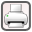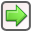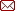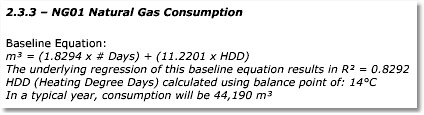﻿ Report Structure > Utility Invoice Analysis > Meter Modeling > Meter Baseline Equation
 Navigation:  Report Structure > Utility Invoice Analysis > Meter Modeling > Meter Baseline Equationrev. 10/29/2010The baseline equation in this example is:

m3 = (1.8294 x # Days) + (11.2201 x HDD14)

If you picked any time period and knew the average (mean) daily temperature for each day in the period, you could calculate the number of heating degree days at a heating balance point of 14ºC.  From there you could use the equation to calculate the expected natural gas consumption for the period.

In this example, the natural gas consumption is heating-sensitive...gas use increases as the outside air temperature declines below 14ºC.  This analysis showed that this meter is not sensitive to cooling load, but if it was cooling-sensitive as well (gas use increased above a particular outside air temperature), there would also be a term for that (e.g. ... + (2.56 x CDD21) ).

Utility consumption can be sensitive to heating, cooling, and up to three additional independent user variables.

The baseline formula is commonly used after conservation projects are implemented, to compare actual utility invoices against what the model would have predicted.  ManagingEnergy  automates these calculations, to factor out independent variables and determine actual energy performance of projects.